Practice Test: Ratio & Proportion- 2

# Practice Test: Ratio & Proportion- 2

Test Description

## 10 Questions MCQ Test CSAT Preparation for UPSC CSE | Practice Test: Ratio & Proportion- 2

Practice Test: Ratio & Proportion- 2 for Banking Exams 2022 is part of CSAT Preparation for UPSC CSE preparation. The Practice Test: Ratio & Proportion- 2 questions and answers have been prepared according to the Banking Exams exam syllabus.The Practice Test: Ratio & Proportion- 2 MCQs are made for Banking Exams 2022 Exam. Find important definitions, questions, notes, meanings, examples, exercises, MCQs and online tests for Practice Test: Ratio & Proportion- 2 below.
Solutions of Practice Test: Ratio & Proportion- 2 questions in English are available as part of our CSAT Preparation for UPSC CSE for Banking Exams & Practice Test: Ratio & Proportion- 2 solutions in Hindi for CSAT Preparation for UPSC CSE course. Download more important topics, notes, lectures and mock test series for Banking Exams Exam by signing up for free. Attempt Practice Test: Ratio & Proportion- 2 | 10 questions in 20 minutes | Mock test for Banking Exams preparation | Free important questions MCQ to study CSAT Preparation for UPSC CSE for Banking Exams Exam | Download free PDF with solutions
 1 Crore+ students have signed up on EduRev. Have you?
Practice Test: Ratio & Proportion- 2 - Question 1

### The ratio of the number of boys and girls in a college is 7 : 8. If the percentage increase in the number of boys and girls be 20% and 10% respectively, what will be the new ratio?

Detailed Solution for Practice Test: Ratio & Proportion- 2 - Question 1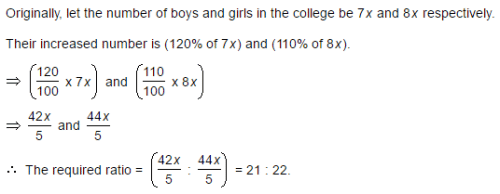Practice Test: Ratio & Proportion- 2 - Question 2

### Salaries of Ravi and Sumit are in the ratio 2 : 3. If the 'salary of each' one of them is increased by Rs. 4000, the new ratio becomes 40 : 57. What is Sumit's salary?

Detailed Solution for Practice Test: Ratio & Proportion- 2 - Question 2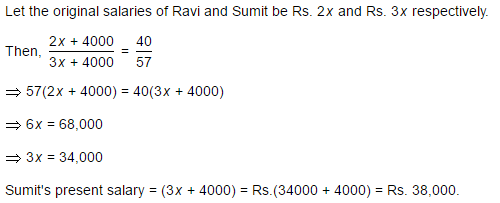Practice Test: Ratio & Proportion- 2 - Question 3

### The salaries A, B, C are in the ratio 2 : 3 : 5. If the increments of 15%, 10% and 20% are allowed respectively in their salaries, then what will be new ratio of their salaries?

Detailed Solution for Practice Test: Ratio & Proportion- 2 - Question 3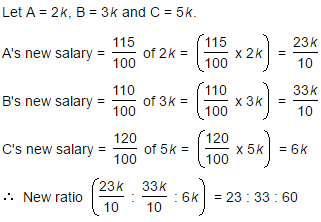Practice Test: Ratio & Proportion- 2 - Question 4

If 40% of a number is equal to two-third of another number, what is the ratio of first number to the second number?

Detailed Solution for Practice Test: Ratio & Proportion- 2 - Question 4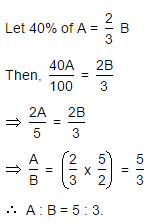Practice Test: Ratio & Proportion- 2 - Question 5

The fourth proportional to 5, 8, 15 is:

Detailed Solution for Practice Test: Ratio & Proportion- 2 - Question 5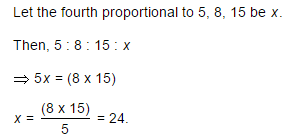Practice Test: Ratio & Proportion- 2 - Question 6

In a bag, there are coins of 25 p, 10 p and 5 p in the ratio of 1 : 2 : 3. If there is Rs. 30 in all, how many 5 p coins are there?

Detailed Solution for Practice Test: Ratio & Proportion- 2 - Question 6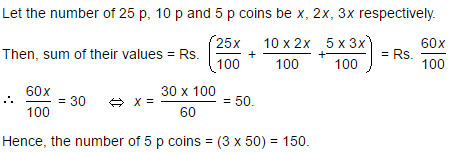Practice Test: Ratio & Proportion- 2 - Question 7

Two number are in the ratio 3 : 5. If 9 is subtracted from each number, the new numbers are in the ratio 12 : 23. The smaller number is:

Detailed Solution for Practice Test: Ratio & Proportion- 2 - Question 7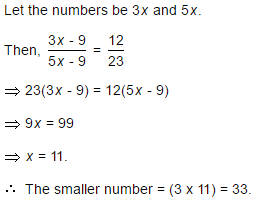Practice Test: Ratio & Proportion- 2 - Question 8

If 0.75 : x :: 5 : 8, then x is equal to:

Detailed Solution for Practice Test: Ratio & Proportion- 2 - Question 8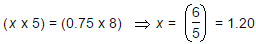Practice Test: Ratio & Proportion- 2 - Question 9

The sum of three numbers is 98. If the ratio of the first to second is 2 :3 and that of the second to the third is 5 : 8, then the second number is:

Detailed Solution for Practice Test: Ratio & Proportion- 2 - Question 9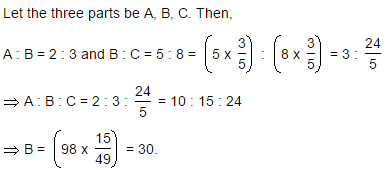Practice Test: Ratio & Proportion- 2 - Question 10

In a garrison of 3600 men, the provisions were sufficient for 20 days at the rate of 1.5 kg per man per day. If x more men joined, the provisions would be sufficient for 12 days at the rate of 2 kg per man per day. Find x.

Detailed Solution for Practice Test: Ratio & Proportion- 2 - Question 10

Let x be the number of new men joined the garrison,
The total quantity of food is = 3600(20) (1.5) kg ----------1
Now the available food will be consumed by (3600 + x) men
(3600 + x) (12) (2) kg  --------------2
1 = 2
Solving both the equations
3600(20) (1.5) = (3600 + x) (12) (2)
108000 = 86400 + 24x
21600 = 24x
X = 900
900 more men joined the garrison.

## CSAT Preparation for UPSC CSE

72 videos|64 docs|92 tests
 Use Code STAYHOME200 and get INR 200 additional OFF Use Coupon Code
Information about Practice Test: Ratio & Proportion- 2 Page
In this test you can find the Exam questions for Practice Test: Ratio & Proportion- 2 solved & explained in the simplest way possible. Besides giving Questions and answers for Practice Test: Ratio & Proportion- 2, EduRev gives you an ample number of Online tests for practice

## CSAT Preparation for UPSC CSE

72 videos|64 docs|92 tests